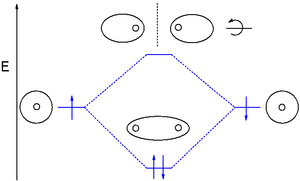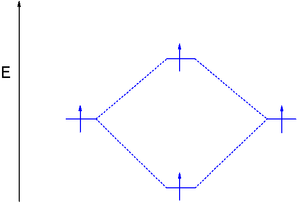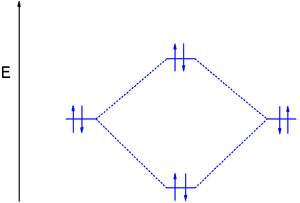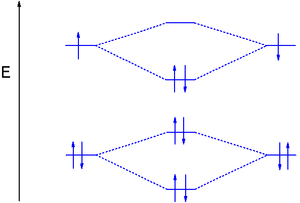## Bond Order

#### Learning Objective

• Calculate a molecule’s bond order given its molecular orbital diagram.

#### Key Points

• Bond order is defined as half the difference between the number of bonding and antibonding electrons.
• Stable bonds have a positive bond order.
• Bond order is an index of bond strength and is used extensively in valence bond theory.

#### Terms

• acetyleneethyne; the simplest alkyne, a hydrocarbon of formula HC≡CH; a colorless gas, with a peculiar, unpleasant odor, formerly used as an illuminating gas but now used in welding and metallurgy
• sigma bonda covalent atomic bond that is rotationally symmetric about its axis
• bond orderthe number of overlapping electron pairs between a pair of atoms
• antibondingan atomic or molecular orbital whose energy increases as its constituent atoms converge, generating a repulsive force that hinders bonding

Bond order is the number of chemical bonds between a pair of atoms; in diatomic nitrogen (N≡N) for example, the bond order is 3, while in acetylene (H−C≡C−H), the bond order between the two carbon atoms is 3 and the C−H bond order is 1. Bond order indicates the stability of a bond. In a more advanced context, bond order does not need to be an integer.

## Bond Order in Molecular Orbital Theory

In molecular orbital theory, bond order is also defined as the difference, divided by two, between the number of bonding and antibonding electrons; this often, but not always, yields the same result. Bond order is also an index of bond strength, and it is used extensively in valence bond theory.

## Dihydrogen (H2)

This MO diagram depicts the molecule H2, with the contributing AOs on the outside sandwiching the MO. The bonding level (lower level) is completely occupied. A bond order of one is obtained by employing the formula above, indicating a stable bond.

$\text{Bond Order} = \frac{2 (\text{bonding electrons})-0(\text{anti-bonding}\ e-)}{2} = 1$Stable dihydrogen moleculeA bond order of one indicates a stable bond.

## Dihydrogen (H2) with an Electron in the Antibonding Orbital

In the second diagram, one of the bonding electrons in H2 is “promoted” by adding energy and placing it in the antibonding level.

$Bond \ Order = \frac{1 (bonding\ electrons)-1(anti-bonding\ e-)}{2} = 0$

The above formula verifies breaking the H2 bond, which in this case gives a bond order of zero. For a bond to be stable, the bond order must be a positive value.Dihydrogen with an electron in the antibonding orbitalBy adding energy to an electon and pushing it to the antibonding orbital, this H2 molecule’s bond order is zero, effectively showing a broken bond.

## Dihelium (He2)

The third diagram hypothesizes the molecule dihelium (He2). A bond order of zero is obtained by placing the available electrons in the bonding and antibonding levels, indicating that dihelium does not exist according to valence bond and bond order theory.

$Bond \ Order = \frac{2 (bonding\ electrons)-2(anti-bonding\ e-)}{2} = 0$

However, removing an electron from the antibonding level produces the molecule He2+, which is stable in the gas phase with a bond order of 0.5.

$Bond \ Order = \frac{2 (bonding\ electrons)-1(anti-bonding\ e-)}{2} = 0.5$The electron configuration of diheliumIf the molecule He2 were to exist, the 4s electrons would have to fully occupy both the bonding and antibonding levels, giving a bond order of zero. Dihelium does not exist.

## Dilithium (Li2)

The last diagram presents the molecule dilithium (Li2). The 1s electrons do not take part in the bonding, but the 2s electrons fill the bonding orbital. The molecule Li2 is a stable molecule in the gas phase, with a bond order of one.

$Bond \ Order = \frac{2 (bonding\ electrons)-0(anti-bonding\ e-)}{2} = 1$The dilithium moleculeWithout the 1s electrons participating in bonding, the p electrons completely fill the bonding orbital; this leaves the antibonding orbital empty and gives a bond order of one, indicating a stable molecule (in this case, in the gas phase).